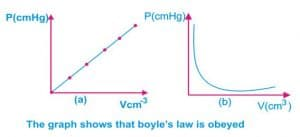Physic Tutorials

Short notes on gas law for UTME

Short notes on gas law for UTME

Boyle’s law

Boyle’s law states that the volume of a fixed mass of gas is inversely proportional to the pressure provided that the temperature remains constant.

If p is the pressure, v the volume and t the temperature in the Kelvin, Then the law can be stated as follows:

P α 1/v   i.e p = k/v (k is constant)

PV = constant (k is constant).

If P1 and V1 are the initial pressure and volume of the gas respectably, and P2 and V2 are the final pressure and volume after the change, then Boyle’s law can be written thus:

P1V1 = P2V2Charles’ law

Charles’ law state that the volume of a fixed mass of gas is directly proportional to its absolute temperature (T) provided the pressure remains constant. Mathematically,

V α T  where V is the volume, T = absolute temp

V = kT(k is constant)

V/T  =k(k= constant)

V1/T1 = V2/T2

where V1 and T1 are the initial volume and temperature and V2 and T2 are the final volume and temperature respectively.

Pressure law

Pressure law or gay Lussa’s law states that the pressure of a fixed mass of gas at constant volume is proportional to its absolute temperature.

Mathematically

P α T

P/T = k(k = constant)

P1/T1 = P2/T2

Where P1 and T1 are initial pressure and Temperature while P2 and T2 are final pressure and temperature respectively

The ideal gas equation (general gas equation)

The ideal gas equation is a combination of the three gas laws i.e. Boyle’s law, Charley’s law and the pressure law.

From Boyle’s law we have PV = constant at constant temperature

From Charles’ law we have V/T = constant at constant temperature

From pressure law we have P/T = constant at constant volume combining these three laws

Therefore PV/T = constant

P1V1/T1 = P2V2/T2

Example

An ideal gas has a pressure of 100cmHg at a temperature of 27 degree when its volume is 120cm3. When the pressure and temperature are increased to 150cmHg and 127 degree respectively. Calculate the new volume of the gas.

Solution

The temperature must be converted to kelvin. 1.e

The first temperature 27 degree = 273+27 = 300 kelvin

The second temperature 127 degree = 273 + 127 = 400 kelvin

P1V1/T1 = P2V2/T2

100*120/300 = 150*V2/400

V2 = (100*120*400)/(300*150) = 106.67cm3

The standard and temperature is 0 degree or 273k while the standard pressure is 760mmHg. The standard volume is 22.4 dm3.

The kinetic theory and its explanation of the gas laws

The kinetic theory of gas is made up of larger number of molecules. These molecules move about in their container randomly with different velocity, colliding with one another and the container walls. As the gas molecules hit the walls of the container and their velocity change as well as their momentum. The wall experience some force as a result of change in momentum of the gas molecule. Therefore, some pressure is exerted on walls of the vessel used by the collision of the molecules, since pressure is force per unit area.

Questions and answers on gas law for UTME

Bolarinwa Olajire

A lecturer, Educationist, PhD student at FUNAAB, and a Blogger.